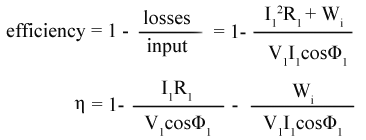There is no machine which has not any loss. The transformer also has some losses. Yes, the Transformer is a very efficient machine than other machines such as motors, and generators. The losses in the transformer are very low. Here you will see all types of Transformer Losses and Efficiency Measurements.

## Transformer Losses

The losses in the transformer which are described in this article are,

• Copper Loss
• Iron Loss
• Eddy Current Loss
• Hysteresis Loss
• Dielectric Loss
• Stray Loss

### Copper Loss in Transformer

Copper loss is related to Resistance and Current. Copper Loss occurs in a transformer due to the resistance of the winding. Transformer windings have some resistance, so when a current flows through the transformer Copper Loss(Cu loss) occurs.
Copper loss is also known as "I squared R Losses".

Generally, the transformer  has two windings primary winding and secondary winding both have their individual resistance, so, therefore, the total copper loss will be,It is clear that the copper loss is proportional to the squared of the current, so as the current depends on the load if the load changes current will change. If current changes Copper Loss also changed. So Copper Loss is a Variable Loss.

#### Effect of Copper Loss in a Transformer

The main effect of Copper Loss in a Transformer is, that it creates heat which may damage the winding.

#### Reducing Copper Loss

1. Copper Loss can be reduced by increasing the cross-sectional area of the conductor.
2. Keeping a constant load current.
3. Making conductors with high-conductivity materials.

### Iron Loss in Transformer

Basically, the Iron loss is a constant loss. Iron loss occurs in the transformer depending on the input voltage and the magnetic properties of the materials used in the Core. The iron loss also depends upon the Frequency.
As iron loss is caused by the alternating flux in the core of the transformer it is also called Core Loss.
The iron loss has two components, Hysteresis Loss and Eddy Current Loss

### Eddy Current Loss in Transformer

In a transformer, when the AC power supply is given, the primary winding creates an alternating flux which is linked with the secondary winding. Thus an EMF is induced in the secondary winding. In the transformer, the Core is used as a path for conducting flux from primary winding to secondary winding.

So the alternating flux is also linked with the steel core which creates an emf in the core. The EMF produced in the core causes to flow a circulating current in the core. This circulating current does not do any useful work, even it produces heat and creates loss which is called Eddy Current Loss.According to the transformer emf equation, the production of flux is dependent upon the input voltage so the Iron Loss or Eddy current loss depends upon the input voltage. As the voltage is always constant so the iron loss or eddy current loss is also constant.

#### Reducing Eddy Current Loss

Eddy current loss can be reduced by laminating the core.

### Hysteresis Loss in Transformer

We know that the Transformer works on an AC supply, so the alternating flux works in the transformer. when alternating flux conducts from primary winding to secondary winding through the core, it creates the reversal of magnetization in the transformer core. This reversal of the magnetization in the transformer core creates a loss which is called Hysteresis Loss
The Hysteresis Loss depends upon the frequency, the value of flux density, grade of the iron core.#### Reducing Hysteresis Loss

Hysteresis Loss can be reduced by using Silicon Steel in the Transformer Core.

### Dielectric Loss in Transformer

The losses in insulating material in the transformer are called Dielectric Loss. Dielectric electric loss occurs in transformer insulating oil and solid insulation between transformer core laminations. Dielectric Loss can create a short circuit, heating inside the transformer.

### Stray Loss in Transformer

All the produced flux in the primary winding is not linked with the secondary winding of the transformer. There is some leakage of flux that occurs in the transformer. So the loss that occurs due to the leakage flux of leakage magnetic field is called Stray Loss.

#### Reducing Stray Loss

Stray loss can be minimized by using core material which has high permeability and low reluctance.

## Measurement of Losses in Transformer

When a transformer is installed in any industry or substation, some tests are performed to determine or measurement of losses, efficiency, and voltage regulation of the Transformer.

### Measurement of Copper Loss by Short Circuit Test

Short Circuit test is performed in the transformer to measure Copper Loss. The short Circuit(S.C) test is also called the Impedance Test. The connection diagram for the short circuit test on the transformer is given below. Short Circuit test is performed on the High Voltage(H.V) side of the transformer.

### Measurement of Iron Loss by Open Circuit Test

An Open Circuit(O.C) test is performed in the transformer to measure Iron Loss. The connection diagram for the open circuit test on the transformer is given below. An open circuit test is performed on the Low Voltage(L.V) side of the transformer.

## Transformer Efficiency

Why a Transformer is a highly efficient machine than other machines?

We know that the transformer is a static device, which does not have any moving parts so there is no mechanical loss occurring in the transformer.
Copper loss in the transformer is less than the other machines because its winding resistance is very low. For these reasons, the transformer has better efficiency.

### The formula for Transformer EfficiencyThe efficiency of a transformer will be maximum when the Iron Loss becomes equal to the Copper Loss.

[ALL] Types of Transformer Losses and Efficiency MeasurementReviewed by Author on April 27, 2019 Rating: 5